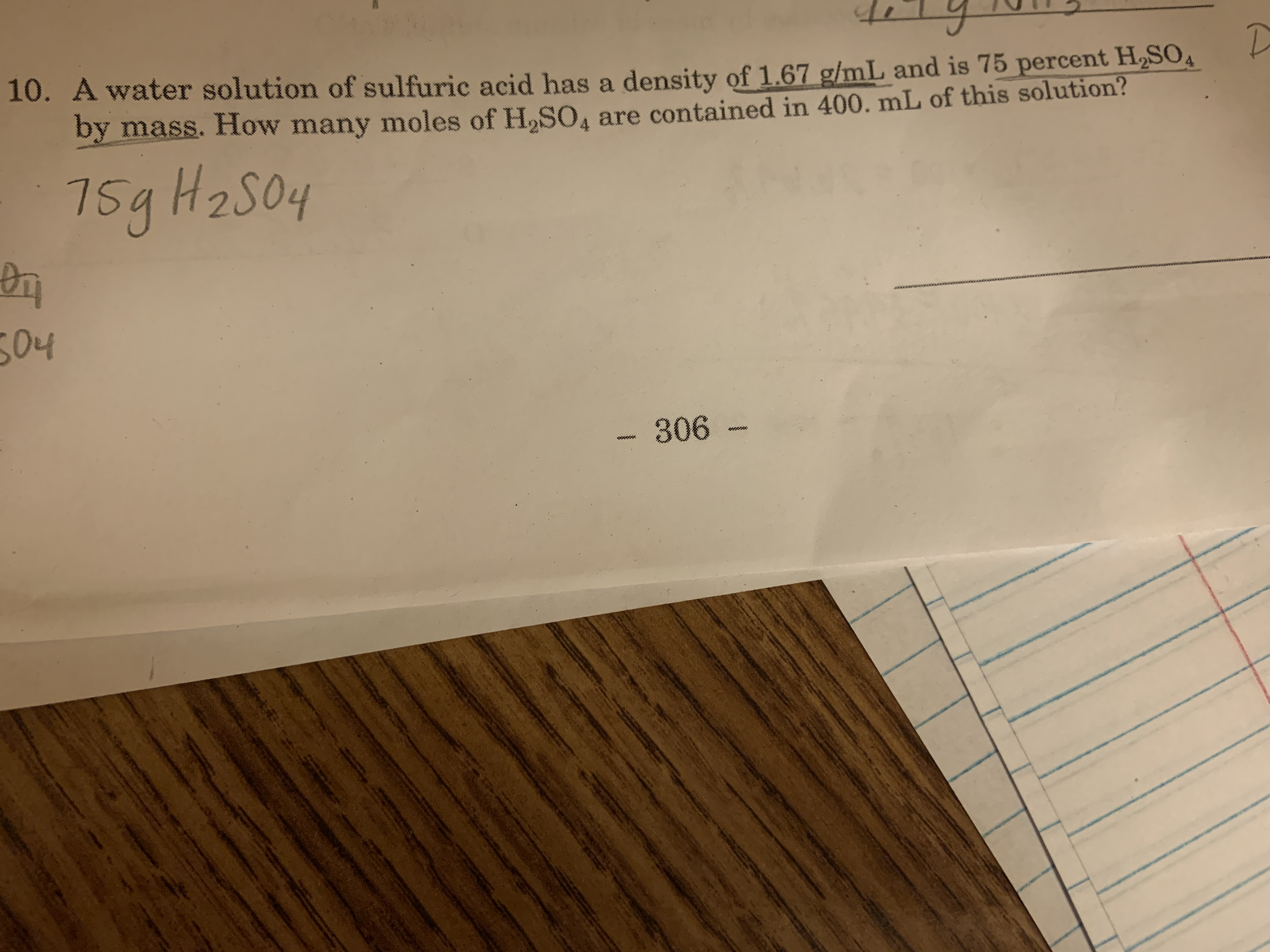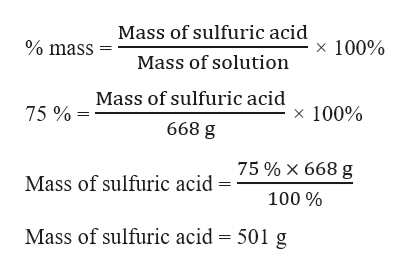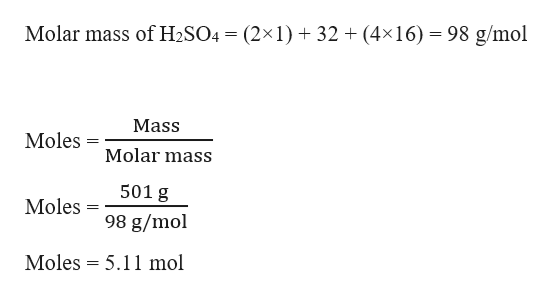# 10. A water solution of sulfuric acid has a density of 1.67 g/mL and is 75 percent HSOby mass. How many moles of H2SO4 are contained in 400. mL of this solution?75g H2soy2S0404306 -

Questionhelp_outlineImage Transcriptionclose10. A water solution of sulfuric acid has a density of 1.67 g/mL and is 75 percent HSO by mass. How many moles of H2SO4 are contained in 400. mL of this solution? 7 5g H2soy 2S04 04 306 - fullscreen
check_circleExpert Solution
Step 1

Given,

Density of solution = 1.67 g/mL

Mass percent of sulfuric acid in the solution = 75 %

Volume of solution = 400 mL

Mass of solution can be calculated as:

Step 2

The mass of sulfuric acid can be calculated using the mass percent formula:help_outlineImage TranscriptioncloseMass of sulfuric acid % mass x 100% Mass of solution Mass of sulfuric acid x 100% 75% 668 g 75% X 668 g Mass of sulfuric acid 100% Mass of sulfuric acid 501 g fullscreen
Step 3

Therefore, moles of sulfuric ac...help_outlineImage TranscriptioncloseMolar mass of H2SO4 (2x1)32 (4x16) 98 g/mol Mass Moles Molar mass 501 g Moles 98 g/mol Moles 5.11 mol fullscreen

### Want to see the full answer?

See Solution

#### Want to see this answer and more?

Solutions are written by subject experts who are available 24/7. Questions are typically answered within 1 hour*

See Solution
*Response times may vary by subject and question
Tagged in

### General Chemistry